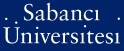# On the minimum distance of cyclic codes

Işık, Leyla (2011) On the minimum distance of cyclic codes. [Thesis]Preview
PDF - Requires a PDF viewer such as GSview, Xpdf or Adobe Acrobat Reader
214Kb

## Abstract

Estimation of the minimum distance of cyclic codes is a classical problem in coding theory. Using the trace representation of cyclic codes and Hilbert's Theorem 90, Wolfmann found a general estimate for the minimum distance of cyclic codes in terms of the number of rational points on certain Artin-Schreier curves. In this thesis, we try to understand if Wolfmann's bound can be improved by modifying equations of the Artin-Schreier curves by the use of monomial and some nonmonomial permutation polynomials. Our experiments show that an improvement is possible in some cases.

Item Type: Thesis Finite fields. -- Cyclic codes. -- Trace representations. -- Permutation polynomials. -- Sonlu cisimler. -- Devirsel kodlar.-- İz gösterimleri. -- Permütasyon polinomları. Q Science > QA Mathematics 21615 IC-Cataloging 14 Jun 2013 15:00 25 Mar 2019 17:05

Repository Staff Only: item control page